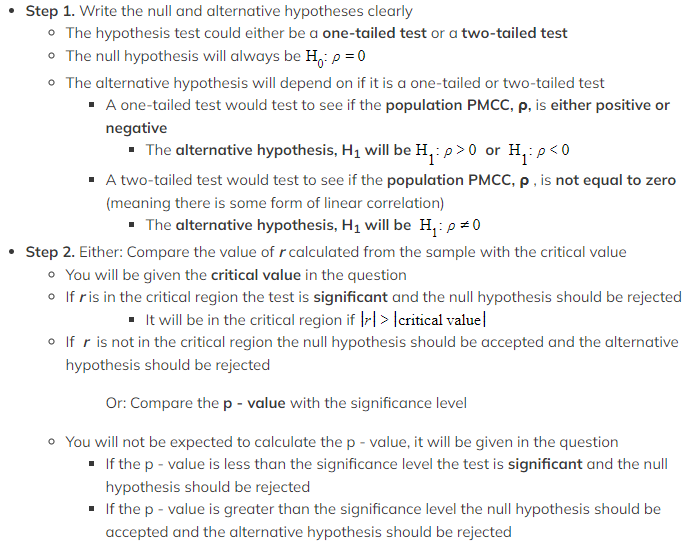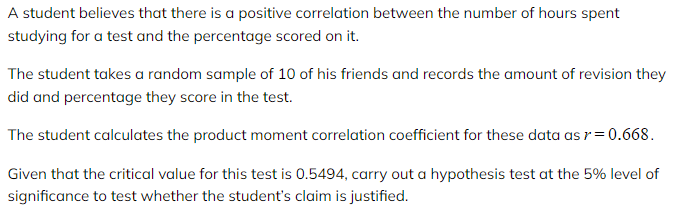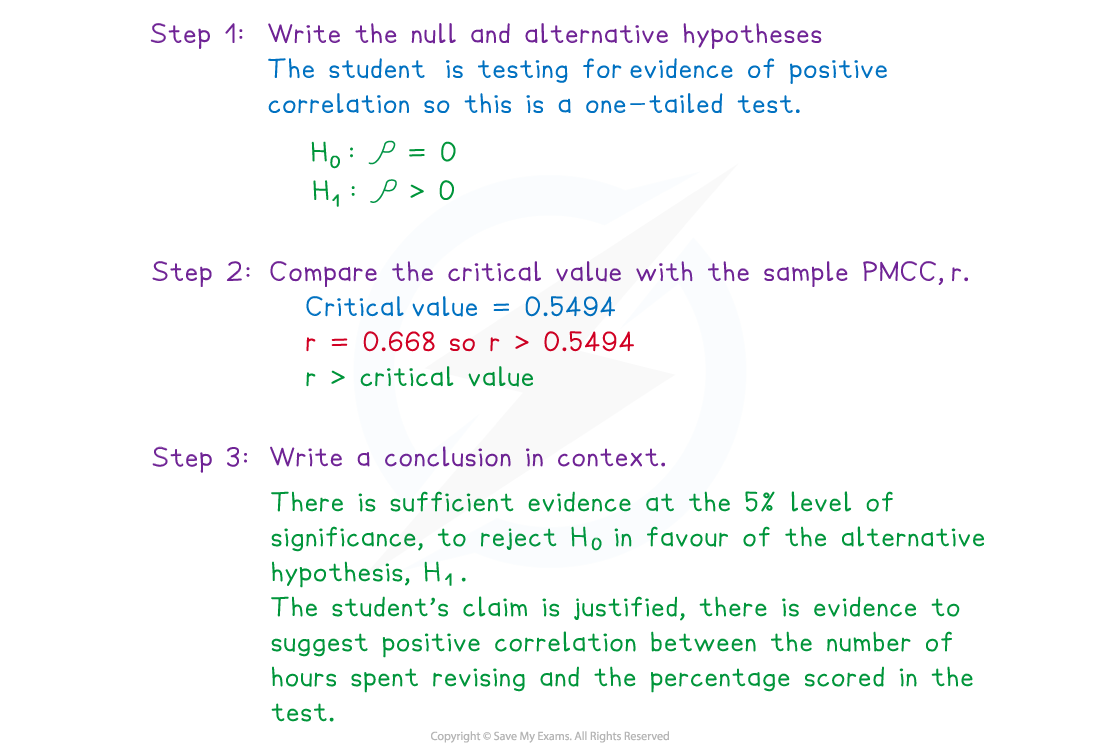# AQA A Level Maths: Statistics复习笔记2.5.2 Hypothesis Testing for Correlation

### Hypothesis Testing for Correlation

You should be familiar with using a hypothesis test to determine bias within probability problems. It is also possible to use a hypothesis test to determine whether a given product moment correlation coefficient calculated from a sample could be representative of the same relationship existing within the whole population.  For full information on hypothesis testing, see the revision notes from section 5.1.1 Hypothesis Testing

#### Why use a hypothesis test?

• In most cases it is too difficult to get the value of the PMCC for a whole population
• This would involve having data on each individual within the whole population
• It is very rare that a statistician would have the time or resources to collect all of that data
• The PMCC for the whole population can instead be estimated using information from a sample taken from the population
•The PMCC for a sample taken from the population is denoted r
• A hypothesis test would be conducted using the value of to r determine whether the population can be said to have positive, negative or zero correlation

#### How is a hypothesis test for correlation carried out?

• Most of the time the hypothesis test will be carried out by using a critical value
• You won't be expected to calculate p-values but you might be given a p-value
•Step 3. Write a conclusion in context
• Use the wording in the question to help you write your conclusion
• If rejecting the null hypothesis your conclusion should state that there is evidence to accept the context of the alternative hypothesis at the level of significance of the test only
• If accepting the null hypothesis your conclusion should state that there is not enough evidence to accept the context of the alternative hypothesis at the level of significance of the test only

#### Worked Example#### Exam Tip

• Make sure you read the question carefully to determine whether the test you are carrying out is for a one-tailed or a two-tailed test and use the level of significance accordingly. Be careful when comparing negative values of r with a negative critical value, it is easy to make an error with negative numbers when in an exam situation.